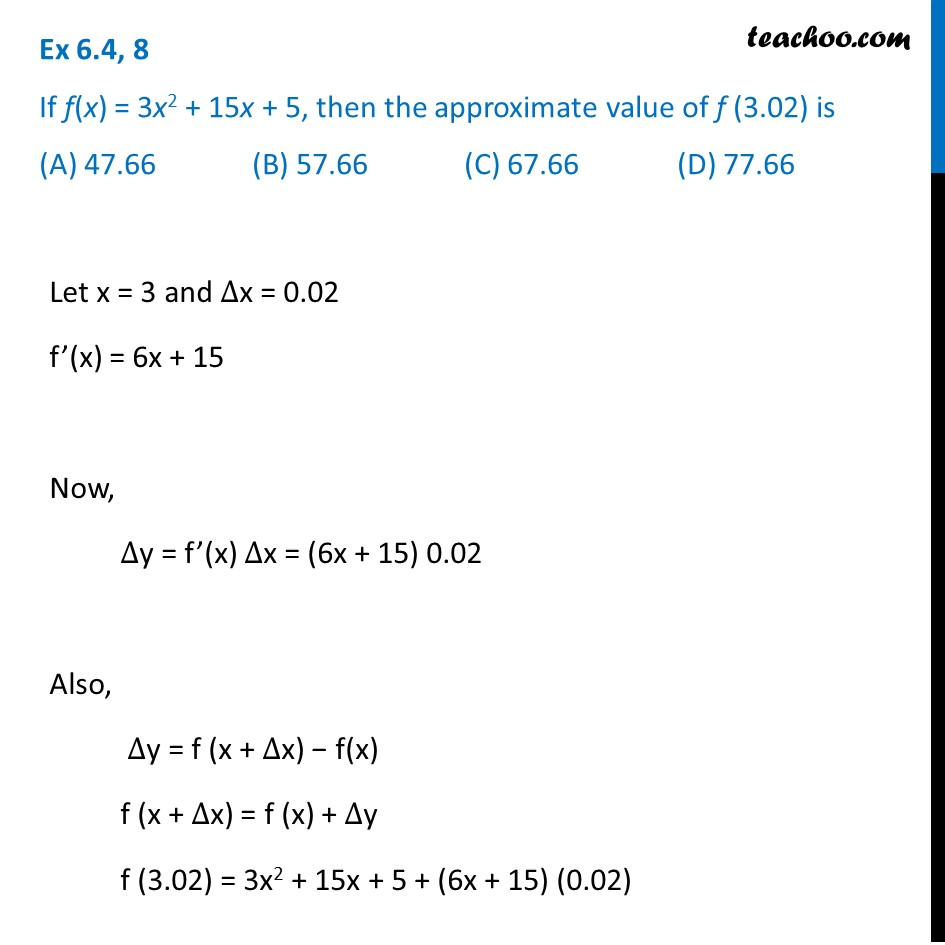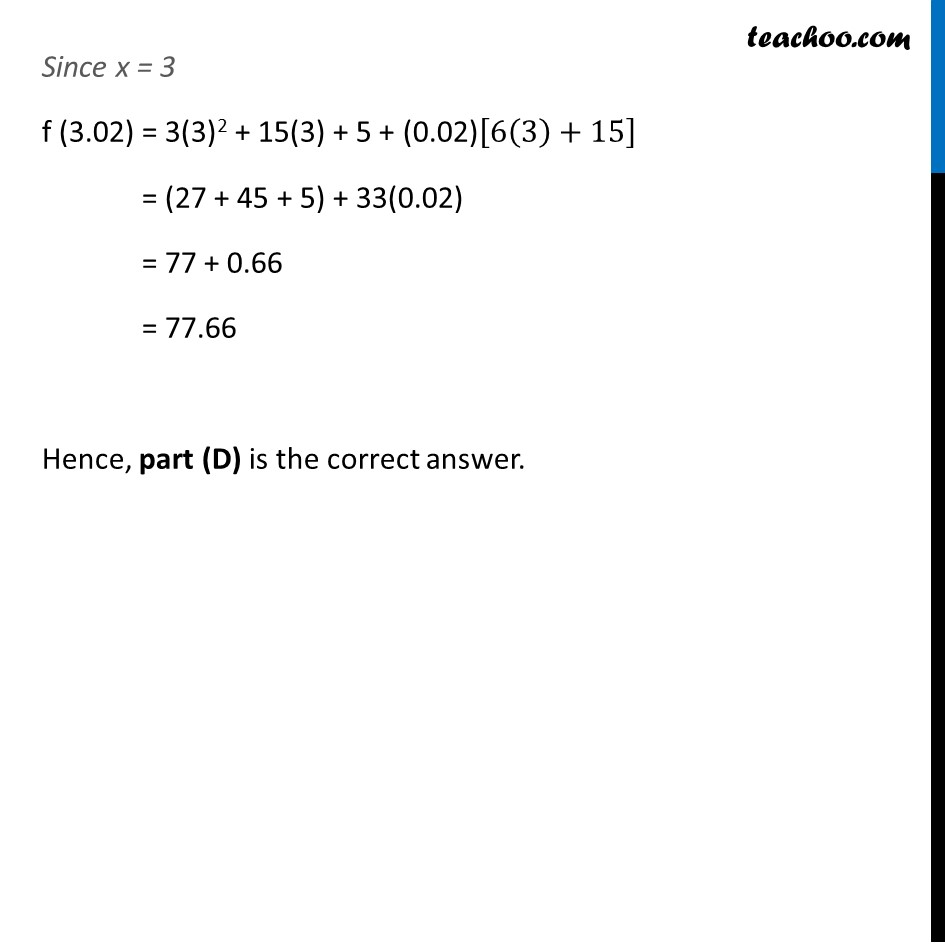Approximations (using Differentiation)

Chapter 6 Class 12 Application of Derivatives
Serial order wiseLearn in your speed, with individual attention - Teachoo Maths 1-on-1 Class

### Transcript

Question 8 If f(x) = 3x2 + 15x + 5, then the approximate value of f (3.02) is (A) 47.66 (B) 57.66 (C) 67.66 (D) 77.66Let x = 3 and ∆x = 0.02 f’(x) = 6x + 15 Now, ∆y = f’(x) ∆x = (6x + 15) 0.02 Also, ∆y = f (x + ∆x) − f(x) f (x + ∆x) = f (x) + ∆y f (3.02) = 3x2 + 15x + 5 + (6x + 15) (0.02) Since x = 3 f (3.02) = 3(3)2 + 15(3) + 5 + (0.02)[6(3)+15] = (27 + 45 + 5) + 33(0.02) = 77 + 0.66 = 77.66 Hence, part (D) is the correct answer.